# All About Multiplication: 10 Worksheets

Multiplying large numbers, decimals and fractions takes serious practise. Help your fifth grader ace his times tables with this collection of multiplication worksheets.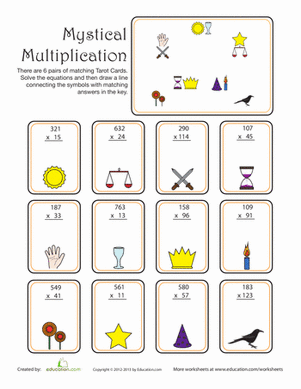### Mystical Multiplication

Add a bit a magic and mystery to your normal maths practices. With mystical multiplication, you'll multiply to find the matching tarot cards!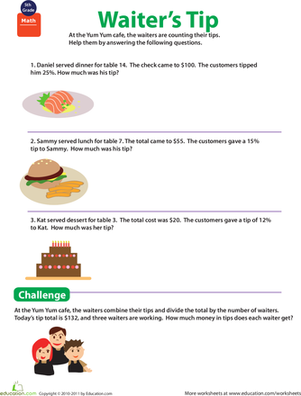### Waiter Tips maths

Can your fifth grader use his percentage and multiplication skills to help these waiters count their tips?### Multiplying Numbers with Decimals

If your child needs a little help with decimal multiplication, here's the worksheet for her.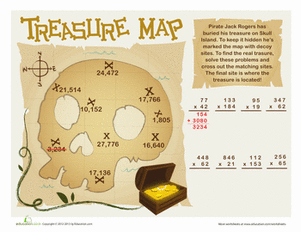### Treasure maths Map

The treasure of notorious Jack Rogers is hidden on this treasure maths map that gives your child fun multiplication practise!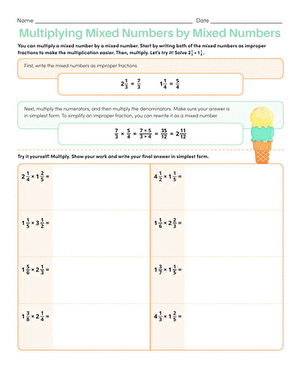### How to Multiply Mixed Numbers by Mixed Numbers

This valuable worksheet teaches your little mathematician how to convert mixed numbers to fractions, and then multiply them.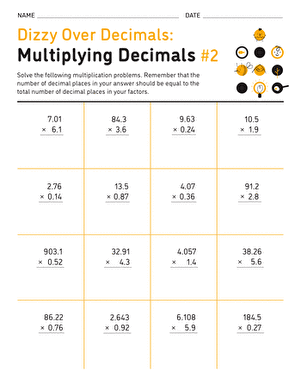### Dizzy Over Decimals: Multiplication #2

Here's a sheet that's sure to give your child all the practise he needs with decimal multiplication.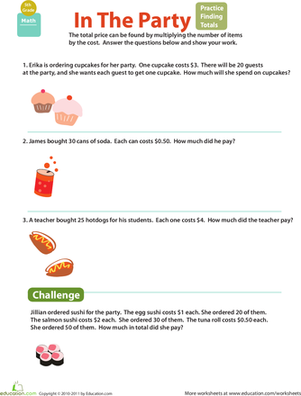### Money maths: practise Finding Totals

Can your fifth grader ring up the total prices? She'll use her multiplication muscles in the process!### How to Multiply Decimals

Use this worksheet to learn all about multiplying decimals.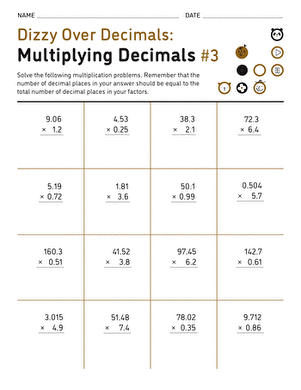### Dizzy Over Decimals: Multiplication #3

This maths practise sheet is chock-full of decimal multiplication problems that are guaranteed to help your child get more comfortable with his schoolwork.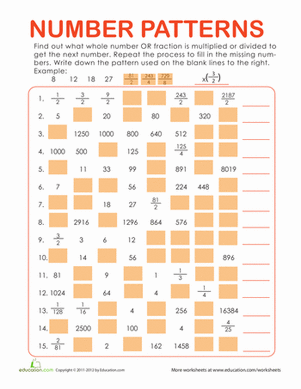### Number Patterns: Multiply or Divide

Finding patterns is key in mathematics. Can you find the patterns here? This worksheet is perfect for extra practise with fractions and times tables.

Create new collection

0

### New Collection>

0Items

What could we do to improve Education.com?